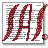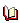﻿ datavis.ca: SAS Graphic Programs and Macros#SAS Graphic Programs and MacrosMichael Friendly
York University

This page provides access to SAS programs and macros for statistical graphics, and related documentation. Some were initially described in The SAS System for Statistical Graphics, First Edition and in Visualizing Categorical Data, however many of these have been updated and extended since their original publication.

Most of them require SAS/GRAPH, SAS/STAT and/or SAS/IML as well as base SAS. See the Usage Notes for local modifications you may have to make at your site and for general descriptions of macro parameter conventions.

A considerable number of additional macros are also included here. Some are described below, but all are now linked on the menu at the top of this page, and under the Macro categories tree.

## Macro categories tree

Click on a folder to expand the subtree. Hover over a macro name for a brief description. Click on a macro name for documentation and source code. Enjoy!In addition, if you use any of these programs in research reports, you are expected to acknowledge this use with an appropriate citation. For macros from The SAS System for Statistical Graphics, First Edition or Visualizing Categorical Data, the following are appropriate references. BibTex versions are here.

Friendly, M. (2000). Visualizing Categorical Data. Cary, NC: SAS Institute. ISBN 1-58025-660-0.
Friendly, M. (1991). SAS System for Statistical Graphics, First Edition. Cary, NC: SAS Institute. ISBN 1-55544-441-5.

For other macros, an appropriate reference has the form:
Friendly, M. (2007). heplots macro: Plot Hypothesis and Error matrices for a MLM. Version 1.1-1 (09 Jan 2007). http://www.datavis.ca/sasmac/heplots.html.

## Some macro descriptions

This section lists just a few of these macros, largely for historical purposes. It will never be updated.boxcox macro
Finds power transformations of the response variable in a regression model (PROC REG) by the Box-Cox method, with graphic display of the maximum likelihood solution, t-values for model effects, and the influence of observations on choice of power.boxglm macro
Finds power transformations of the response variable in a general linear model (PROC GLM) by the Box-Cox method.caliscmp macro
Compare model fits from PROC CALIS. The CALISCMP macro extracts goodness of fit statistics from the RAM data sets produced in a series of models fit to a given data set using PROC CALIS. The COMPARE= parameter allows pairs of nested models to be tested.canplot macro
Canonical discriminant structure plot Displays the observations in a dataset in the space of the two canonical variables which discriminate most among groups. Also plots class means on the canonical variates, confidence circles for those means, and variable vectors showing the correlations of variables with the canonical variates..coplot macro
Constructs a conditioning plot - plots of Y * X | Z, showing how the relationship between X and Y depends on Z.cpplot macro
Plots of Mallow's C(p) and related statistics for model selection in linear modelscorrgram macro
The CORRGRAM macro produces a schematic display of a correlation matrix, called a correlogram. In this display, variables are permuted so that ``similar'' variables are positioned adjacently, and cells of a matrix are shaded or filled to show the correlation value.cqplot macro
The cqplot macro produces quantile-quantile comparison plots for multivariate normal data (based on squared Mahalanobis distances from the centroid) or for other data which should follow a Chi-square distribution, together with estimated confidence bands.csmpower macro
The csmpower macro carries out retrospective or prospective power computations for covariance structure models using the method of MacCallum, Browne and Sugawara (1996).datachk macro
The datachk macro performs basic data screening/checking on numeric variables in a dataset, and is designed to give a compact overview of many variables.dummy macro
The dummy macro creates dummy (0/1) variables to represent the levels of a discrete variable in a regression model. If the original variable has c levels, (c-1) new variables are produced.faces macro
Draws (possibly asymmetric) faces to represent multivariate observations.fpower macro
Power computations for ANOVA designsgtree macro
Draw a tree dendrogram from PROC CLUSTER/VARLCUS outputhemat macro
Plot Hypothesis and Error matrices for all pairs of variablesheplot macro
Plot Hypothesis and Error matrices for a bivariate MANOVA effectinflogis macro
Produces an influence plot for a logistic regression model. The plot shows a measure of badness of fit for a given case (DIFDEV or DIFCHISQ) vs. the fitted probability (PRED) or leverage (HAT), using an influence measure (C or CBAR) as the size of a bubble.inflplot macro
Produces an influence plot for a regression model -- a plot of studentized residuals vs. leverage (hat-value), using COOK's D or DFFITS as the size of a bubble symbol.label macro
Constructs an Annotate data set to label points in a 2- or 3-D scatter plot.jitter macro
The JITTER macro adds a small amount of noise to numeric variables, usually to avoid overplotting for discrete data.meanplot macro
The meanplot macro produces 1-way, 2-way, or 3-way plots of means for a factorial design with any number of factor variables.missrc macro
The missrc macro estimates cell probabilities in an n-way table with ignorable missing data (missing completely at random [MCAR], or missing at random [MAR]) on the table variables. The results are equivalent to the use of the EM algorithm.mpower macro
The mpower macro performs retrospective power analysis for a multivariate PROC GLM analysis. This treats the sample means as if they were population means, determines an effect size, and calculates the power for specified effects.orpoly macro
Constructs orthogonal polyomial contrasts (allowing unequal spacing | N)panels macro
The panels macro constructs a template in which to replay a series of panels, assumed all the same size, in a rectangular array of R rows and C columns.power macro
The power macro provides power calculations for general linear models.powerrxc macro
The powerrxc macro computes approximate power for Pearson and Likelihood Ratio Chi-square tests of independence in PROC FREQ.resline macro
Fits a resistant line to X-Y data and determines transformations to make the relation linear.robcov macro
The ROBCOV macro calculates robust estimates of the mean vector, variance-covariance matrix and/or correlation matrix for a multivariate sample. It also calculates classical estimates of multivariate (Mahalanobis) distance, and robust estimates of multivariate distance which ignore potential outliers. Optionally, it produces a plot of robust vs. classical distances.rpower macro
Retrospective power analysis for univariate GLMs.rsqdelta macro
Compute R-square change and F-statistics in regression.scale macro
Scale a set of variables to a given range.scatter macro
Scatterplot matrix with SAS/INSIGHT. The scatter macro uses SAS/INSIGHT to produce a scatterplot matrix for two or more variables, showing all pairwise plots. If observations are classified by a grouping variable, colors and symbols may be assigned automatically to the observations in different groups.splot macro
Draws low-res (printer) schematic plots (boxplots) for one or more variables.sprdplot macro
The sprdplot macro produces a spread-level plot to determine if a simple power transformation can equalize within-group variance of a response variable in a dataset classified by one or more classification variables.stat2dat macro
The stat2dat macro takes a dataset containing summary statistics (N, mean, std dev) for a between groups design and produce a dataset from which PROC GLM can be run, producing an ANOVA summary table equivalent to that calculated from the raw data.sunplot macro
Sunflower plot for X-Y data. The sunflower plot displays a bivariate dataset using "sunflower symbols" to show the number of observations in the neighborhood of each XY point.symbox macro
Displays boxplots of a single variable raised to various powers in side-by-side boxplots as an aid to finding a power transformation to symmetry.